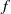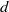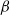Hostname: page-component-758b78586c-kdfvs Total loading time: 0 Render date: 2023-11-28T17:25:04.041Z Has data issue: false Feature Flags: { "corePageComponentGetUserInfoFromSharedSession": true, "coreDisableEcommerce": false, "useRatesEcommerce": true } hasContentIssue false

# Gibbs and equilibrium measures for some families of subshifts

Published online by Cambridge University Press:  16 May 2012

## Abstract

For subshifts of finite type (SFTs), any equilibrium measure is Gibbs, as long as$f$ has$d$-summable variation. This is a theorem of Lanford and Ruelle. Conversely, a theorem of Dobrušin states that for strongly irreducible subshifts, shift-invariant Gibbs measures are equilibrium measures. Here we prove a generalization of the Lanford–Ruelle theorem: for all subshifts, any equilibrium measure for a function with$d$-summable variation is ‘topologically Gibbs’. This is a relaxed notion which coincides with the usual notion of a Gibbs measure for SFTs. In the second part of the paper, we study Gibbs and equilibrium measures for some interesting families of subshifts:$\beta$-shifts, Dyck shifts and Kalikow-type shifts (defined below). In all of these cases, a Lanford–Ruelle-type theorem holds. For each of these families, we provide a specific proof of the result.

Type
Research Article
Information
Ergodic Theory and Dynamical Systems , June 2013 , pp. 934 - 953

## Access options

Get access to the full version of this content by using one of the access options below. (Log in options will check for institutional or personal access. Content may require purchase if you do not have access.)

## References

Aaronson, J. and Nakada, H.. Exchangeable, Gibbs and equilibrium measures for Markov subshifts. Ergod. Th. & Dynam. Sys. 27(2) (2007), 321339.Google Scholar
Bowen, R.. Some systems with unique equilibrium states. Math. Syst. Theory 8(3) (1974/75), 193202.Google Scholar
Burton, R. and Steif, J. E.. Non-uniqueness of measures of maximal entropy for subshifts of finite type. Ergod. Th. & Dynam. Sys. 14(2) (1994), 213235.Google Scholar
Dobrušin, R. L.. Gibbsian random fields for lattice systems with pairwise interactions. Funkcional. Anal. i Priložen. 2(4) (1968), 3143.Google Scholar
Feldman, J. and Moore, C. C.. Ergodic equivalence relations, cohomology, and von Neumann algebras. I. Trans. Amer. Math. Soc. 234(2) (1977), 289324.Google Scholar
Hamachi, T. and Inoue, K.. Embedding of shifts of finite type into the Dyck shift. Monatsh. Math. 145(2) (2005), 107129.Google Scholar
Kalikow, S. A..$T, T^{-1}$ transformation is not loosely Bernoulli. Ann. of Math. (2) 115(2) (1982), 393409.Google Scholar
Krieger, W.. On the uniqueness of the equilibrium state. Math. Syst. Theory 8(2) (1974/75), 97104.Google Scholar
Krieger, W.. On dimension functions and topological Markov chains. Invent. Math. 56(3) (1980), 239250.Google Scholar
Krieger, W. and Matsumoto, K.. A lambda-graph system for the Dyck shift and its$K$-groups. Doc. Math. 8 (2003), 7996 (electronic).Google Scholar
Lanford, O. E. and Robinson, D. W.. Statistical mechanics of quantum spin systems. III. Comm. Math. Phys. 9 (1968), 327338.Google Scholar
Lanford, O. E. and Ruelle, D.. Observables at infinity and states with short range correlations in statistical mechanics. Comm. Math. Phys. 13 (1969), 194215.Google Scholar
Marcus, B. and Newhouse, S.. Measures of maximal entropy for a class of skew products. Ergodic Theory (Proc. Conf., Math. Forschungsinst., Oberwolfach, 1978) (Lecture Notes in Mathematics, 729). Springer, Berlin, 1979, pp. 105125.Google Scholar
Meyerovitch, T.. Tail invariant measures of the Dyck shift. Israel J. Math. 163 (2008), 6183.Google Scholar
Parry, W.. On the$\beta$-expansions of real numbers. Acta Math. Acad. Sci. Hungar. 11 (1960), 401416.Google Scholar
Petersen, K. and Schmidt, K.. Symmetric Gibbs measures. Trans. Amer. Math. Soc. 349(7) (1997), 27752811.Google Scholar
Ruelle, D.. Thermodynamic Formalism, 2nd edn(Cambridge Mathematical Library). Cambridge University Press, Cambridge, 2004.Google Scholar
Schmeling, J.. Symbolic dynamics for$\beta$-shifts and self-normal numbers. Ergod. Th. & Dynam. Sys. 17(3) (1997), 675694.Google Scholar
Schmidt, K.. Invariant cocycles, random tilings and the super-$K$ and strong Markov properties. Trans. Amer. Math. Soc. 349(7) (1997), 28132825.Google Scholar
Schmidt, K.. Algebraic coding of expansive group automorphisms and two-sided beta-shifts. Monatsh. Math. 129(1) (2000), 3761.Google Scholar
Walters, P.. Ruelle’s operator theorem and$g$-measures. Trans. Amer. Math. Soc. 214 (1975), 375387.Google Scholar
Walters, P.. A variational principle for the pressure of continuous transformations. Amer. J. Math. 97(4) (1975), 937971.Google Scholar
Walters, P.. Equilibrium states for$\beta$-transformations and related transformations. Math. Z. 159(1) (1978), 6588.Google Scholar